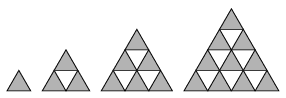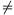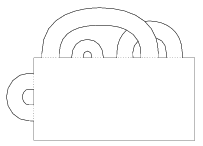Mathematical and Physical Journal
for High Schools
Issued by the MATFUND Foundation
 Already signed up? New to KöMaL?

# KöMaL Problems in Mathematics, April 2007

Show/hide problems of signs:## Problems with sign 'C'

Deadline expired on May 15, 2007.

C. 895. The series of diagrams shown is made up of more and more dark regular triangles. The series is continued to the n-th diagram according to the rule that can be observed. How many dark triangles are used altogether?(5 pont)

solution (in Hungarian), statistics

C. 896. The vertices of a triangle ABC in the coordinate plane are A(0,4), B(3,0), C(c,6). The area of the triangle is 7. Find c, given that 0<c<3.

(5 pont)

solution (in Hungarian), statistics

C. 897. The acute angle of a rhombus of unit sides is 60o. How many circles are there, such that the vertices of the rhombus are equidistant from the circumference of the circle? What are the radii of the circles?

(5 pont)

solution (in Hungarian), statistics

C. 898. The length of the sides of the regular triangle ABC is 6 cm. A bug starts at the vertex C of the triangle and crawls uniformly towards vertex A at a speed of 4 mm/s. At the same time, another bug starts from vertex B and crawls towards vertex C at a speed of 3 mm/s. How long does it take for the distance between the bugs to reach the smallest value, and what is the smallest distance?

(5 pont)

solution (in Hungarian), statistics

C. 899. For what values of the real parameter v do the following simultaneous equations have no solution?

x+y+z=v,    x+vy+z=v,    x+y+v2z=v2.

(5 pont)

solution (in Hungarian), statistics## Problems with sign 'B'

Deadline expired on May 15, 2007.

B. 3992. A few players are fighting a paintball battle. At a certain time instant, the distances between the players are pairwise different. Everyone shoots at the player closest to him. At most how many players may shoot at the same player?

(4 pont)

solution (in Hungarian), statistics

B. 3993. In a sequence of real numbers, the sum every 5 consecutive terms is positive, and the sum of every 7 consecutive terms is negative. How long may be the sequence?

(4 pont)

solution (in Hungarian), statistics

B. 3994. Find all non-negative integers n for which there are non-negative numbers a and b, such that n2=a+b és n3=a2+b2.

(3 pont)

solution (in Hungarian), statistics

B. 3995. A, B, C, D are collinear points in this order. Draw the regular triangles ABE and CDF on one side of the line. Let G denote the intersection of the circles ACE and BDF on the same side of the line ABCD. Prove that.

(4 pont)

solution (in Hungarian), statistics

B. 3996. We have a rectangular sheet of paper. We want to make polygons of 20 sides from it according to the following rule: In each step, we choose a piece of paper (initially, this must be the original sheet) and cut it in two along a straight line. Continuing the procedure what is the minimum number of cuts needed to get one hundred pieces 20-sided polygons?

Competition problem from Germany

(5 pont)

solution (in Hungarian), statistics

B. 3997. Prove that if the product of the real numbers x, y, z is 1, then

x4+y4+z4+x2y2+y2z2+z2x22(x+y+z).

(4 pont)

solution (in Hungarian), statistics

B. 3998. The vertex P of a rectangular block with edges a, b, c is selected. Consider the plane passing through the vertices adjacent to P. Denote the distance of the plane from P is m. Prove that(4 pont)

solution (in Hungarian), statistics

B. 3999. A regular dodecahedron is inscribed in a unit sphere and vectors are drawn from the centre of the sphere to the vertices. What is the sum of these vectors?

(4 pont)

solution (in Hungarian), statistics

B. 4000. Find the smallest possible value of x2+y2, given that x and y are real numbers, x0 and xy(x2-y2)=x2+y2.

Competition problem from Britain

(5 pont)

solution (in Hungarian), statistics

B. 4001. Xenia, Yvette and Zoe are friends. On a certain day, each of them makes a one-hour visit in the same internet café that is open from 12 noon to 8 pm. Each visits at a random time, independently of the others. What is the probability that they all meet there?

(5 pont)

solution (in Hungarian), statistics## Problems with sign 'A'

Deadline expired on May 15, 2007.

A. 425. Let n2 and letbe positive real numbers such that a1+...+an=x1+...+xn=1. Prove thatPolish competition problem

(5 pont)

statistics

A. 426. For arbitrary positive numbers a, b, c, compute the radius of the greatest circle contained by the ellipsoidCzech competition problem

(5 pont)

statistics

A. 427. We have a sheet of paper with 2007 handles'' - long strips, glued to the sheet at the ends. The handles are allowed to cross each other but they are never twisted. Prove that the surface, consisting of the paper sheet and the handles, has at least two boundary loops. (For example, the configuration in the Figure has three boundary loops.)(5 pont)

statistics